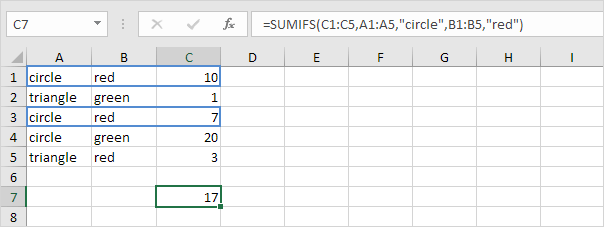# Count and Sum Functions

The most used functions in Excel are the functions that count and sum. You can count and sum based on one criteria or multiple criteria.

### Count

To count the number of cells that contain numbers, use the COUNT function.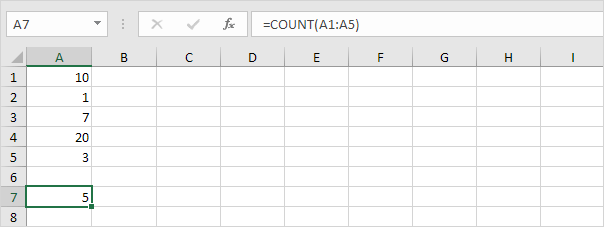Note: to count blank and nonblank cells in Excel, use COUNTBLANK and COUNTA.

### Countif

To count cells based on one criteria (for example, greater than 9), use the following COUNTIF function.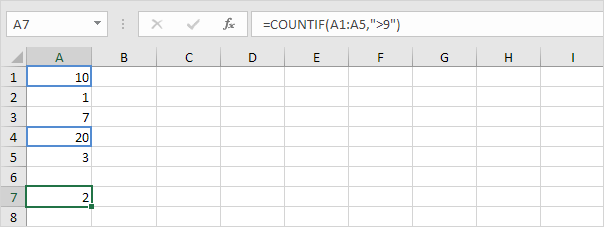### Countifs

To count rows based on multiple criteria (for example, green and greater than 9), use the following COUNTIFS function.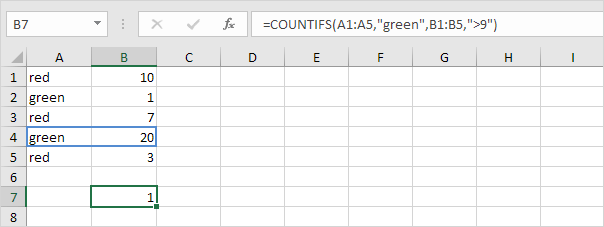### Sum

To sum a range of cells, use the SUM function.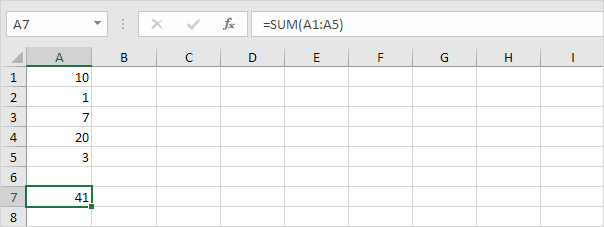### Sumif

To sum cells based on one criteria (for example, greater than 9), use the following SUMIF function (two arguments).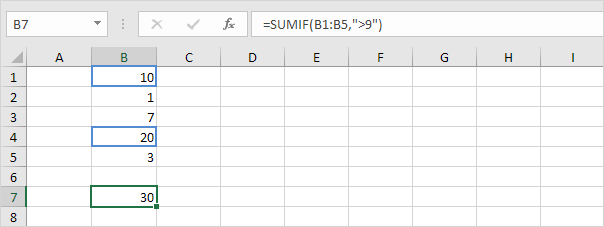To sum cells based on one criteria (for example, green), use the following SUMIF function (three arguments, last argument is the range to sum).